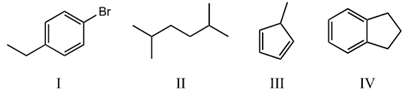# Problem: Which compound below would give rise to 4 signals in the proton NMR spectrum and 6 signals in the carbon NMR spectrum? (Assume you can separate and see all peaks.) A) I B) II C) III D) IV E) More than one of the above.

###### FREE Expert Solution
80% (342 ratings)
###### Problem Details

Which compound below would give rise to 4 signals in the proton NMR spectrum and 6 signals in the carbon NMR spectrum? (Assume you can separate and see all peaks.)

A) I

B) II

C) III

D) IV

E) More than one of the above.Frequently Asked Questions

What scientific concept do you need to know in order to solve this problem?

Our tutors have indicated that to solve this problem you will need to apply the 1H NMR: Number of Signals concept. You can view video lessons to learn 1H NMR: Number of Signals. Or if you need more 1H NMR: Number of Signals practice, you can also practice 1H NMR: Number of Signals practice problems.

What is the difficulty of this problem?

Our tutors rated the difficulty ofWhich compound below would give rise to 4 signals in the pro...as medium difficulty.

How long does this problem take to solve?

Our expert Organic tutor, Jonathan took 4 minutes and 17 seconds to solve this problem. You can follow their steps in the video explanation above.

What professor is this problem relevant for?

Based on our data, we think this problem is relevant for Professor West's class at FAU.